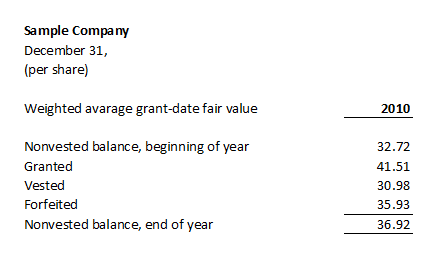Concept Arrangement Patterns (Metapatterns) Visual Summary

Visual image
A hierarchy information model denotes a hierarchy of concepts with no numeric relations. If no numeric relations exist, then the information model of the component is a hierarchy. Basically, anything can be modelled as a hierarchy. It is the addition of additional relations, typically mathematical computations, which turns a hierarchy into some other metapattern.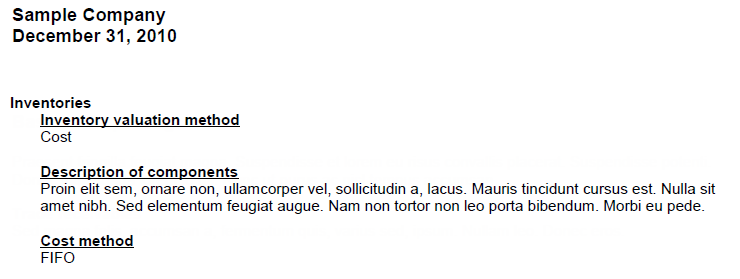A roll up information model computes a total from a set of other concepts. This information model is commonly referred to a roll up, or by the equation A + B = C. All concepts involved in this information model have the same set of characteristics and all must be numeric.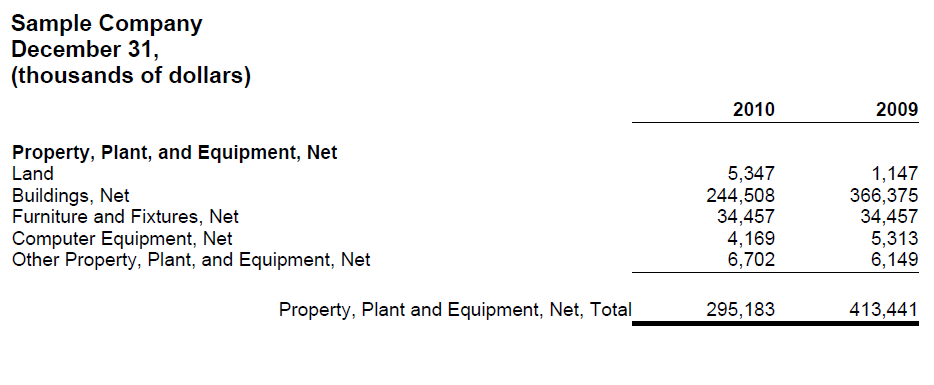A roll forward information model reconciles the balance of a concept between two points in time. This information model is commonly referred to a roll forward or movement analysis or by the equation: beginning balance + changes = ending balance. In this equation period [Axis] is as of two different points in time and the changes occur during the period between those two points in time.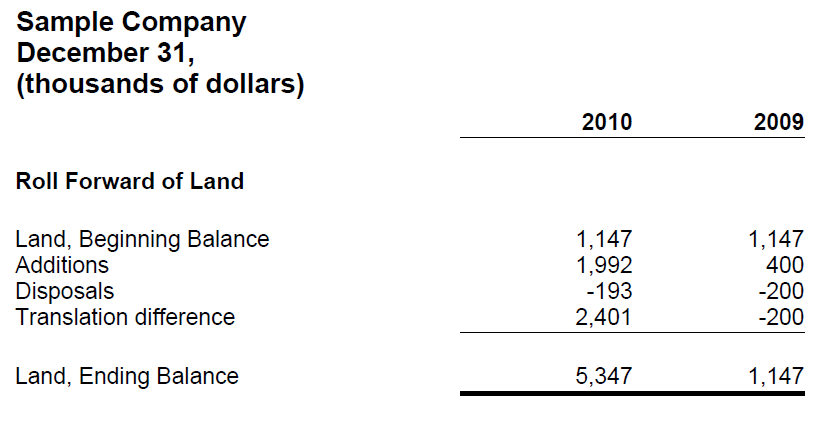A compound fact information model is characterized by the fact that for some set of concepts expressed within some information model; that information model can be expressed over some characteristic expressed as an [Axis]. Basically, it is the [Axis] which provides additional information which makes each information model unique. For example, the salary information for the directors of an entity is a compound fact. The salary information is made up of salary, bonuses, director fees and such information must be associated with a specific director to be meaningful and to distinguish, say, one salary from another salary.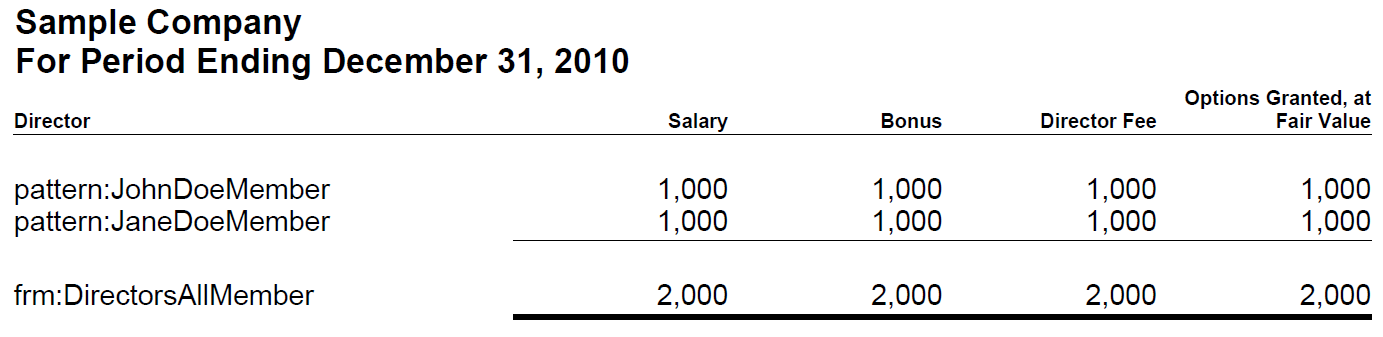An adjustment information model reconciles an originally stated balance to a restated balance, the adjustment being the total change, between two different report dates. An adjustment is similar to a roll forward in that it is a reconciliation, however rather than the period [Axis] changing; it is the Report Date [Axis] which changes: originally reported balance + adjustment = restated balance.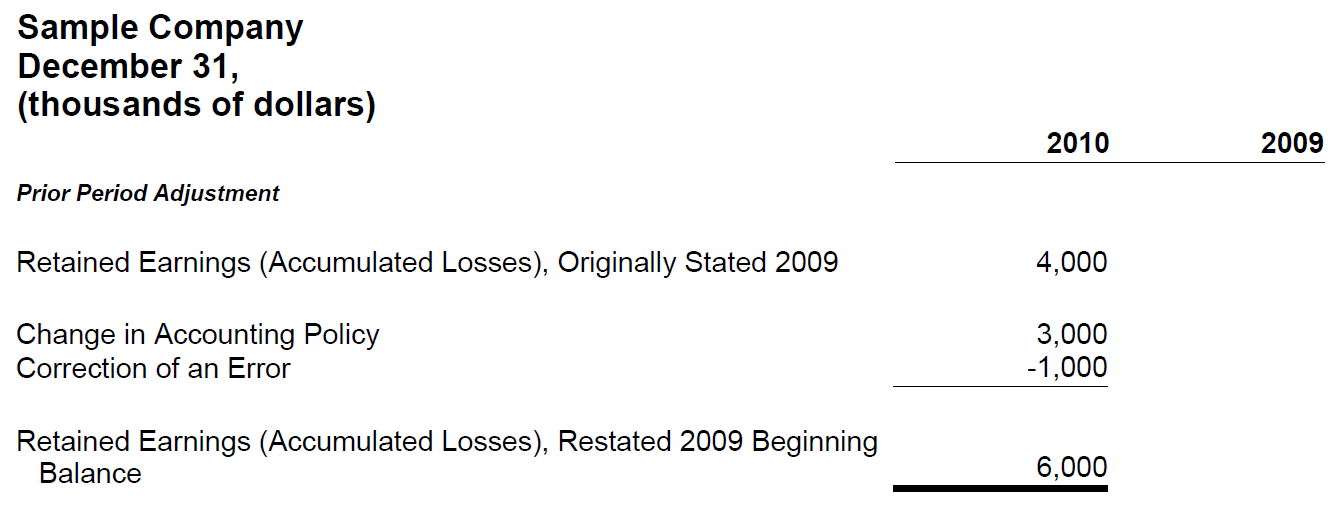A variance information model reconciles some reporting scenario with another reporting scenario, the variance between reporting scenarios being the variance or changes. For example, a sales analysis which reconciles the concept sales for the reporting scenarios of actual and budgeted is a variance. The equation in this case is: actual - budget = variance. But a variance could take other forms such as a variance from forecast, variance from plan, etc.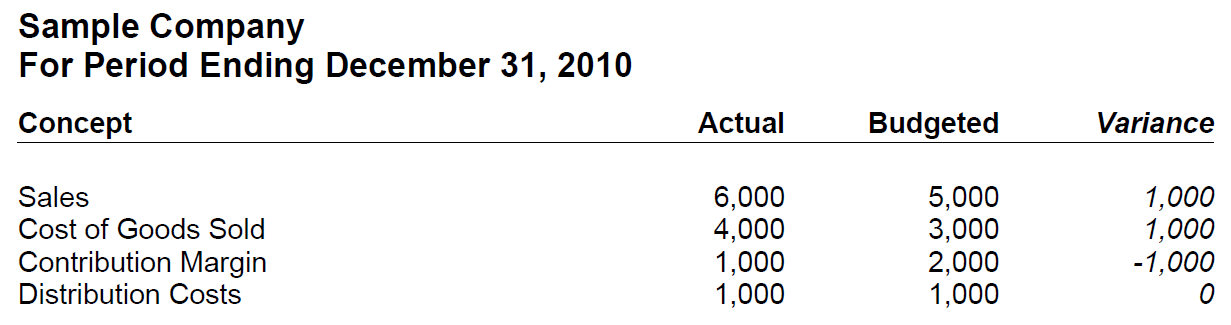A complex computation information model can be thought of as a hierarchy plus a set of commutations between different concepts within that hierarchy which are more challenging to model than a roll up or roll forward. The type of computations can vary significantly, thus the challenging in modelling. For example, the computation of earnings per share is a complex computation.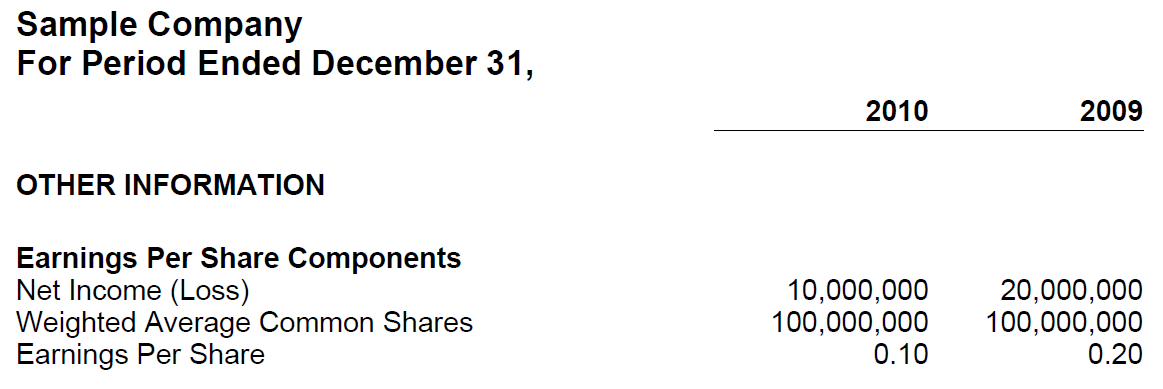A text block information model is an information model which contains, by definition, only one concept and that concept expresses what amounts to a narrative or prose as escaped HTML within that one concept. For example, the narrative associated with a set of accounting policies expressed as a list or a table presentation format is a text block. As there is only one concept, there can be no relations within the information model.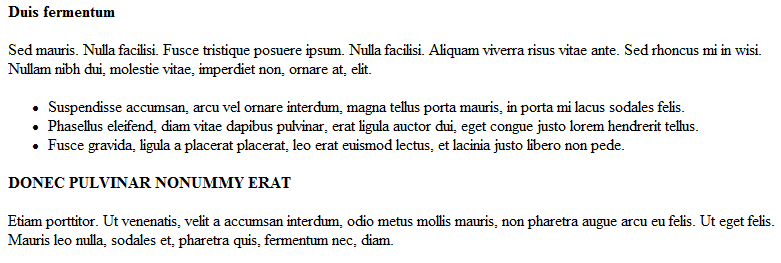A grid information model is a pseudo metapattern which uses the presentation characteristics of the columns and rows of a table to model information. Because the grid models presentation information and not business semantics, it cannot be considered a metapattern. However, the grid is included in this list because the US GAAP Taxonomy uses a grid information model to model the statement of changes in equity.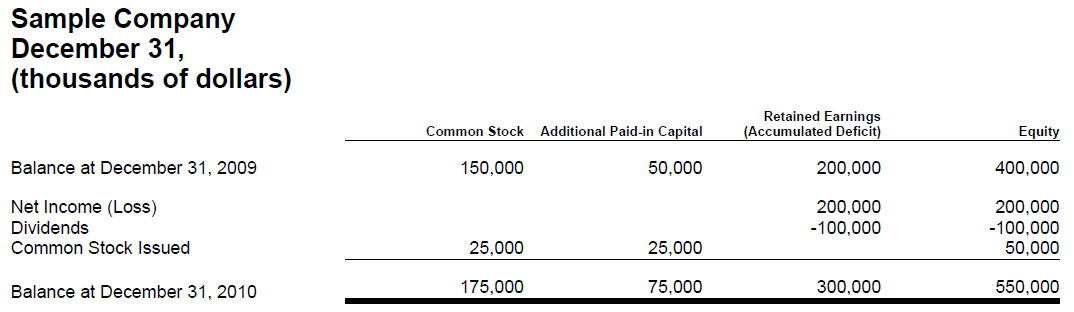A roll forward info is similar to a roll forward in that it has a beginning and ending balance; however, the numbers do not roll forward. The pattern is used to disclose the weighted average price rather than an amount. The data type is always num:perShareItemType.